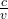Question

TheAurora Borealisis a night display in the Northern latitudes caused by ionizing radiation interacting with the Earth’s magnetic field and the upper atmosphere. The distinctive green color is caused by the interaction of the radiation with oxygen and has a frequency of 5.38 x 1014units. What is the wavelength of this light?

1.niczorrrr

The wavelength of the light is 557.2 x 10⁻⁻⁹nm

Explanation:

The wavelength of a wave is the distance between adjacent troughs and crests while the frequency of a wave is the number of completed cycles that pass a given per unit time

Electromagnetic radiation, such as light is usually described n terms of its frequency and wavelength

The equation relating the three quantities of speed of light, frequency and wavelength is as follows

Speed of light, c = Frequency of the light wave, ν × Wavelength of the light, λ

That is c = ν × λ

Where c =  299792458 m/s, v = 5.38 x 10¹⁴ Hz

Therefore the wavelength == 5.572 x 10⁻⁷ m 557.2 x 10⁻⁻⁹nm